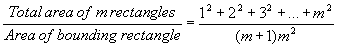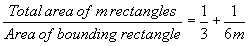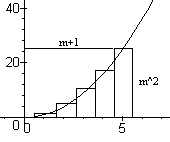# How did Cavalieri get his formula for the area underneath a parabola?

• B
I know he had this ratio:But how did he get this:?

•Delta2

## Answers and Replies•Delta2
Mark44
Mentor
I know he had this ratio:
View attachment 272042
But how did he get this:
View attachment 272043 ?
The result uses a formula for the sum of squares.
$$\sum_{k=1}^m k^2 = \frac{m(m + 1)(2m + 1)}6$$
Replace ##1^2 + 2^2 + \dots + m^2## in your first equation by the above, and you will get the result shown in the second equation.

•Charles Link, Delta2 and erocored